## 3.4.1 A Geometrical Probability Interpretation of PDF's

Somewhat surprisingly, the assumption of spatially uniform and independent points appears in virtually every probability density function that we confront. We can understand this by considering an arbitrary random variable X with pdf fx(x), -< x < +(Figure 3.24a). Instead of viewing the "area under the curve" fx(x) as representing probability, we can view that region as a joint sample space for random variables (X, Y) that are uniformly distributed over the sample space (Figure 3.24b). Random variable X, as before, assumes experimental values -< x < +, and for random variable Y we have 0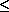y+. Since the area under the curve fx(x) is 1, the joint pdf fx,y(x, y) has height equal to I over the sample space. Given any particular value of X, say X = x, we have 0{Y | X = x}fx(x); in this case {Y| X = x) is uniformly distributed over (0, fx(x)]. This must be an acceptable interpretation since, as before,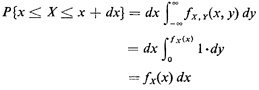Now successive independent experiments that yield values for the random variable X can be viewed as successive independent experiments in which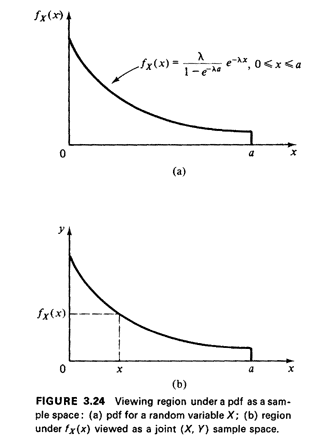(X,Y) are uniformly distributed over this (X, Y) sample space, and in which we are only interested in the experimental value of X. This "geometrical probability" interpretation for fx(x) will be of value in our work. Suppose thatfx(x) is strictly monotonically decreasing with x. Then there exists an inverse function gy(y) = fx-1 (y) which can be viewed as the pdf for the random variable Y. The monotonicity assumption is true for the example of Figure 3.24, and a simple logarithmic transformation shows thatfor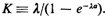. This function, which is simply fx(x) "flipped on its side and turned around" is shown in Figure 3.25. [Note that no scaling is required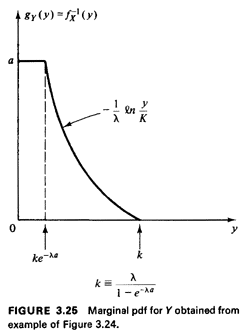since, as mentioned before, the "area of the sample space" in this case must be one because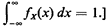The same reasoning can be applied in reverse. That is, we may, in fact, have a spatial situation involving uniformly independently distributed points over some region and we may require the probability laws of the random variables X and Y. Since few geographical regions have area specified to equal unity, care must be taken to scale appropriately the marginal pdf's for X and Y so that they integrate to 1.

Example 9: Triangular Service Area

Suppose that we have a triangular service region of area 1/2 ab serviced by a single facility at the southwest corner of the region (Figure 3.26). Suppose that demands for service are distributed uniformly throughout the region, and either the facility dispatches someone to the demand site or the needer of service travels to the facility. If the needer of service is located at (X, Y), where (X, Y) is uniformly distributed over the region, then the travel distance is X + Y = D.

Utilizing the "geometrical probability" interpretation for pdf's, by inspection we obtain the marginal pdf's for X and Y, both triangular, as shown in Figure 3.26. From earlier work the mean travel distance is found to be E[D] = E[X + Y] = E[X] + E[ Y] = 1/3(a + b) (3.59)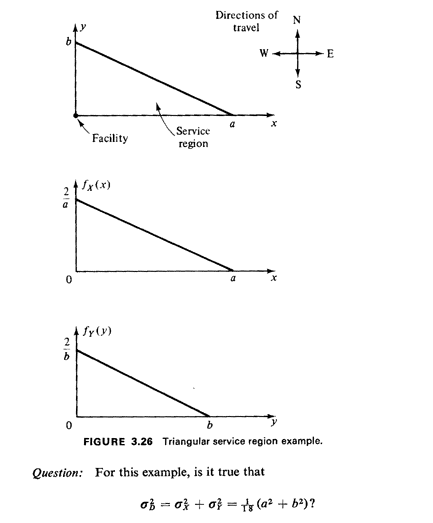Why or why not?

Further work: See Section 3.7.3 and Problems 3.30 and 3.31.